# 海底管道斜裂纹关键因素敏感性分析The Key Factors’ Sensitivity Analysis of Inclined Crack in Submarine Pipeline

• 全文下载: PDF(8842KB)    PP.225-233   DOI: 10.12677/IJM.2017.64023
• 下载量: 667  浏览量: 1,512

Inclined crack is one of the primary factors which results in accident of submarine pipeline. Based on the fracture mechanics theory, we take the numerical method to establish the pipeline model boundary conditions. Then according to the environmental conditions loading environmental load, and calculated the stress intensity factor K, from the pipeline pressure, wall thickness, crack length, pipe diameter, diameter and wall thickness ratio and so on, we also analyzed its sensitivity to pipeline strength. These have a certain foundation effect on the subsequent in-depth study of the pipe with oblique crack pressure.

1. 引言

2. 管道模型参数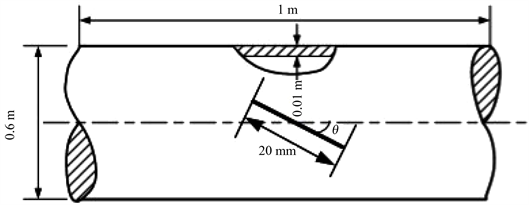Figure 1. Geometric model of pressure piping inclined crack

3. 有限元建模

4. 模型载荷施加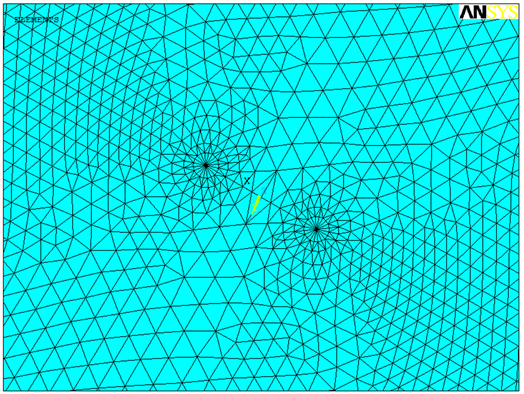Figure 2. The meshing of tip oblique crack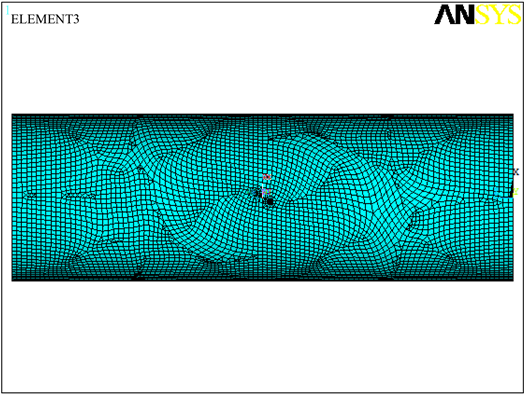Figure 3. Overall grid partition of pressure piping inclined crack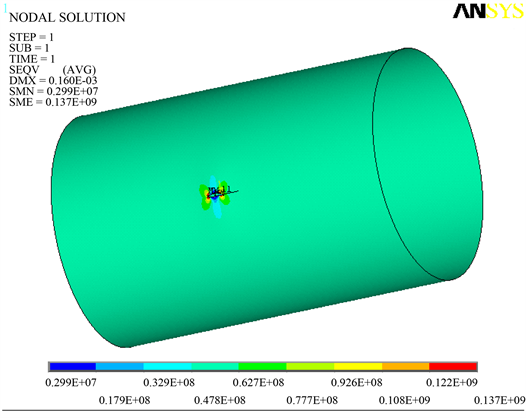Figure 4. Overall stress condition of pressure piping

5. 斜裂纹应力强度因子变化规律Figure 5. Stress condition of tip oblique crack

5.1. 管道内压P的影响

5.2. 管道壁厚t的影响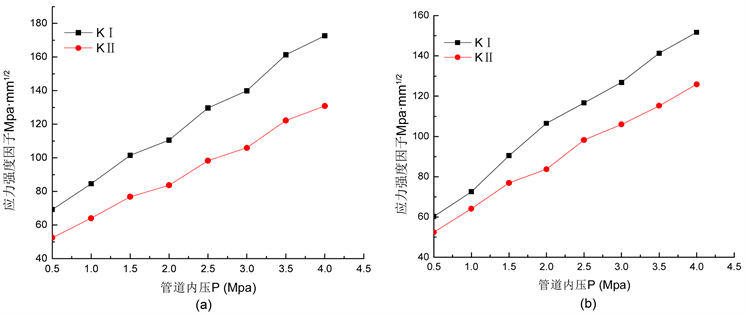Figure 6. Relationship curves of K and pipeline internal pressure P. (a) t = 10 mm; (b) t = 15 mm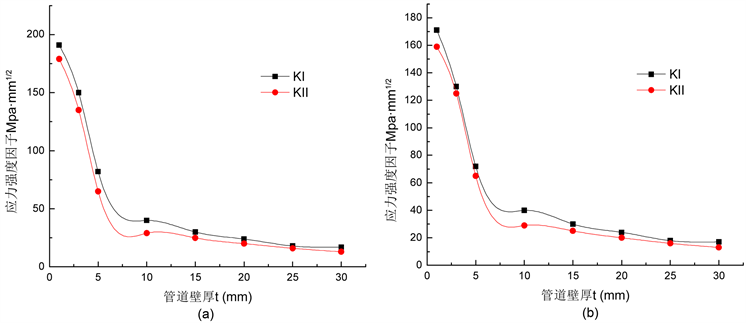Figure 7. Relationship curves of K and pipe wall thickness t. (a) a = 10 mm; (b) a = 20 mm

5.3. 半裂纹长度a的影响

5.4. 管道直径D的影响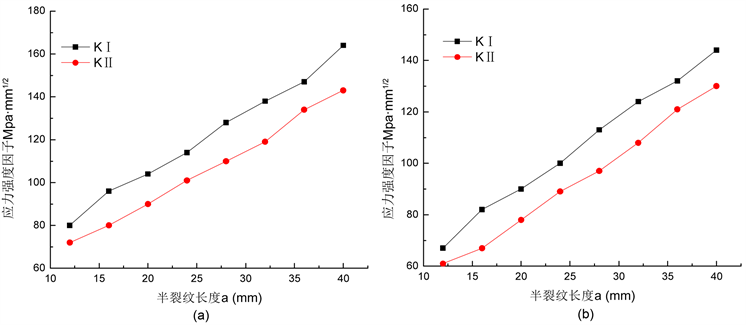Figure 8. The relationship between K and semi-crack length a. (a) t = 10 mm; (b) t = 15 mm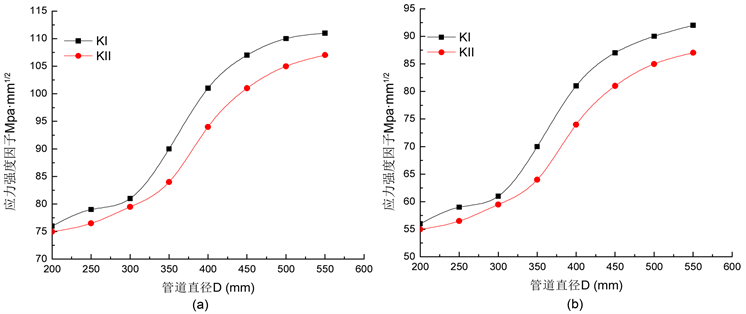Figure 9. The relationship between K and semi-crack length D. (a) a = 10 mm; (b) a = 20 mm

5.5. 裂纹夹角θ的影响

5.6. 管道直径与壁厚比值的影响

6. 结论

1) 在线弹性条件下建立了含有斜裂纹压力管道的有限元模型，在内压载荷条件下求出应力强度因子K，分析了不同因对其影响规律。

2) 在管道直径相同的前提下，管径与壁厚的比值越大(即壁厚越小)，应力强度因子KI和KII的值越Figure 10. The relation curve of K and crack angle θ. (a) a = 10 mm; (b) a = 20 mm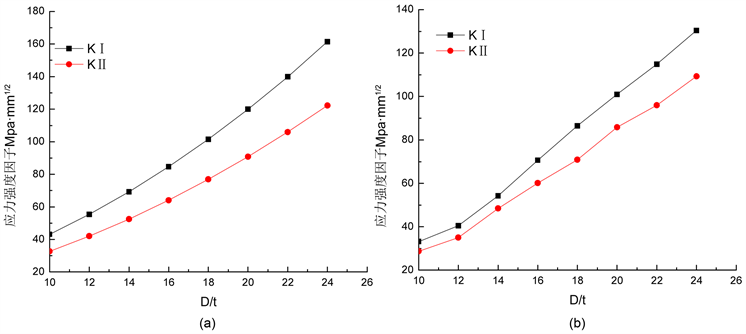Figure 11. The relationship between K and pipe diameter and wall thickness ratio D/t. (a) t = 10 mm; (b) t = 15 mm

3) 本文的分析结果填补了应力强度因子KI和KII的计算空缺，增补了部分形状因子FI和FII的数据库，对后续含斜裂纹压力管道的深入研究奠定了一定基础，计算得到的规律和拟合的相关公式在工程实际中有着很强的应用价值。

  曹宇光, 孔谦, 田凯, 等. 基于有限元模拟的压力管道轴向裂纹应力强度因子计算[J]. 石油矿场机械, 2010, 39(8): 1-7.  Chan, S.K., Tuba, I.S. and Wilson, W.K. (1970) On the Finite Element Method in Linear Fracture Mechanics. Engineering Fracture Mechanics, 2, 1-17.  赵建生. 断裂力学及断裂物理[M]. 武汉: 华中科技大学出版社, 2003.  仇仲翼. 应力强度因子手册[M]. 北京: 科学出版杜, 1993.  范天佑. 断裂理论基础[M]. 北京: 科学出版社, 2003.  沈成康. 断裂力学[M]. 上海: 同济大学出版社, 1996.  马德林. 受内压厚壁圆筒中裂纹尖端应力强度因子[J]. 兵器材料科学与工程, 1985, 8(10): 5-12.  易当祥, 刘春和, 张仕念, 等. 圆管内表面周向三维裂纹应力强度因子的新解法[J]. 飞机设计, 2007, 27(1): 11-15, 26.